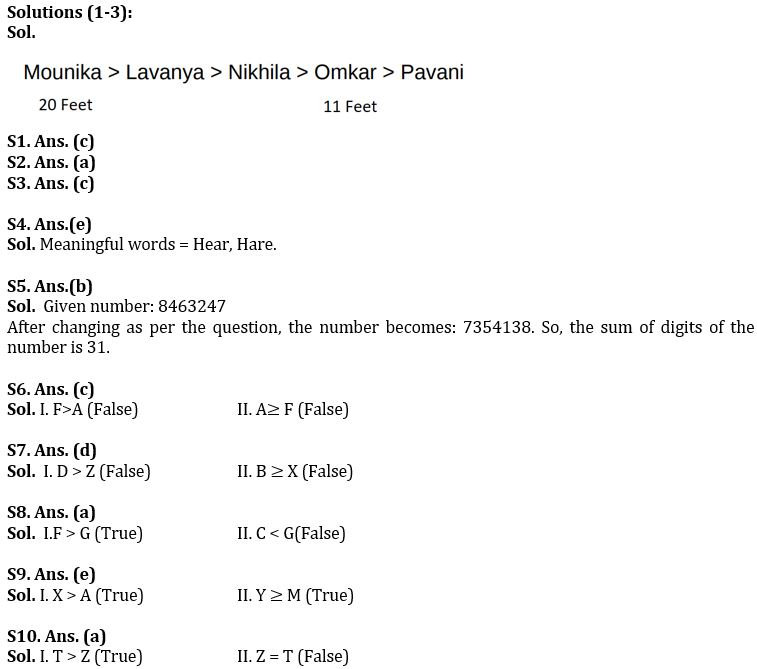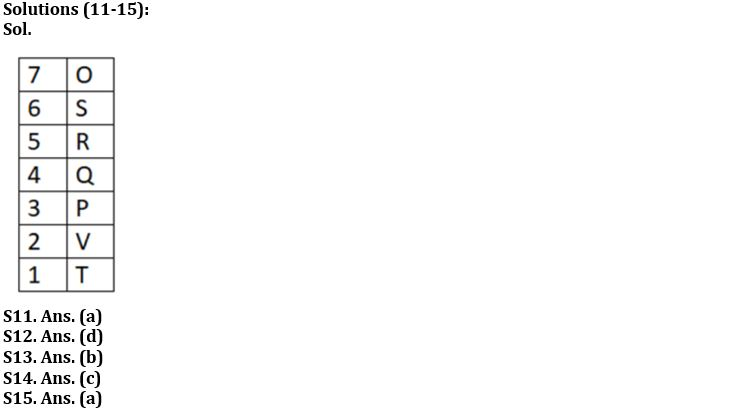Latest Banking jobs   »

# Reasoning Ability Quiz For IBPS Clerk/PO Prelims 2022- 13th August

Directions (1-3): Study the following information carefully and answer the questions given below:
There are five students – Lavanya, Mounika, Nikhila, Omkar and Pavani – each of them having different height. Lavanya is shorter than only Mounika. Omkar is shorter than Nikhila. Omkar is taller than Pavani. The height of the tallest student is 20 feet. The height of the second shortest student is 11 feet.

Q1. What may be the height of Pavani?
(a) 13 feet
(b) 15 feet
(c) 9 feet
(d) 12 feet
(e) 14 feet

Q2. What may be the height of the third tallest student?
(a) 13 feet
(b) 10 feet
(c) 21 feet
(c) 9 feet
(d) 11 feet

Q3. Who is the second shortest student?
(a) Mounika
(b) Nikhila.
(c) Omkar
(d) Lavanya
(e) Cannot be determined

Q4. If it is possible to make a meaningful word from the 1st, 6th, 7th and 9th letters of the word HANDBRAKE, then which will be the last letter of that word? Mark N if no such word can be formed, X if more than one such word can be formed.
(a) H
(b) R
(c) E
(d) N
(e) X

Q5. If each of the odd digits in the number 8463247 is increased by ‘1’ and each of the even digits is decreased by ‘1’, then which of the following will be the sum of the digits of the number?
(a) 38
(b) 31
(c) 27
(d) 29
(e) None of the above

Directions (6-10): In these questions, relationship between different elements is show in the statements. The statements are followed by conclusions. Study the conclusions based on the given statements and select the appropriate answer:
(a) If only conclusion I follows.
(b) If only conclusion II follows.
(c) If either conclusion I or II follows
(d) If neither conclusion I nor II follows.
(e) If both conclusions I and II follow.

Q6. Statements: D > A, F = E ≤B, D>B, C<B
Conclusions I. F>A
II. A≥ F

Q7. Statements: A > Z < B ≥ C > X < D
Conclusions I. D > Z
II. B ≥ X

Q8. Statements: A > B, C ≤B, D < E = B, E < F, G ≤ B
Conclusions I.F > G
II. C < G

Q9. Statements: X > Y ≥ Z = M > A ≥B
Conclusions I. X > A
II. Y ≥ M

Q10. Statements: P < Q > R = Z < S ≤ X < T
Conclusions I. T > Z
II. Z = T

Directions (11-15): Study the following data carefully and answer the questions accordingly.
Seven people live in a seven-story building. The ground floor is numbered 1 and the topmost floor is numbered 7. Q lives above V. R does not live at the bottom. One person lives between P and T. S lives immediately above R. O does not live near to P. P does not live near to S, who does not live on the top. Three people live between T and R.

Q11. Who lives at the bottom?
(a) T
(b) O
(c) V
(d) P
(e) None of these

Q12. How many of them live between S and V?
(a) Two
(b) Four
(c) Five
(d) Three
(e) None of these

Q13. Who lives on the fourth floor?
(a) P
(b) Q
(c) V
(d) O
(e) None of these

Q14. Find the odd one.
(a) T
(b) R
(c) Q
(d) O
(e) P

Q15. How many of them live above R?
(a) Two
(b) Four
(c) Five
(d) Three
(e) None of these

Solutions#### Congratulations!Download Hindu Review of October 2021: Free PDF